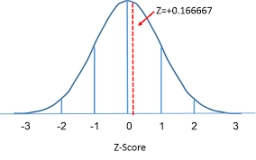# Z score transformation

Suppose a distribution has a mean µ = 8 and standard deviation σ = 4. What is the value of x if it is z = +1.50?

x =  14

### Step-by-step explanation:Did you find an error or inaccuracy? Feel free to write us. Thank you!

Tips for related online calculators
Looking for help with calculating arithmetic mean?
Looking for a statistical calculator?
Looking for a standard deviation calculator?

#### Grade of the word problem:

We encourage you to watch this tutorial video on this math problem: